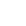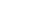# Tags

JavaScriptA singly linked list is a data structure which represents a series of nodes where each node points to the next node in the list. A doubly linked list, in contrast, has nodes which point to the element preceding and following it.

Unlike an array, a linked list doesn’t provide constant-time access to specific indices within the list. So if you need the third element in the list, you have to iterate past the first and second nodes to reach it.

One benefit of a linked list is the ability to add and remove items from the beginning and end of the list in constant time.

These are popular data structures to be questioned about during a technical interviews, so let’s jump right in.

A singly-linked list may be LIFO (last-in-first-out) or FIFO (first-in-first-out). If the list is using the LIFO method, the nodes will be added to and deleted from the same end. If it’s using FIFO, nodes will be added to one end and deleted from the opposite end.

Additionally, the linked list may be sorted. This means that as each node is added to the list, it’s placed into its appropriate spot relative to the other nodes.

## NodeA node has two pieces of information:

• A pointer, or reference, to the next item in the list (for a singly linked list)
• The value of the node

For our node, we’ll just create a function which takes a value, and returns an object with the two values above: a pointer to the next node and the value of the node. Note that we’re able to just declare `value` instead of `value: value`. This is because the variables have the same name. You can learn more about the object property shorthand here.

``````function Node(value) {
return {
value,
next: null
}
}
``````

## NodeList

Now, let’s delve into the NodeList class. This is just that: a list of nodes.Our node list will contain five methods:

• `push(value)`: Pushes a value on to the end of the linked list
• `pop()`: Pops off the last value from the list
• `get(index)`: Returns an item from a given index
• `delete(index)`: Deletes an item from a given index
• `isEmpty()`: Returns a boolean indicating whether the list is empty
• `printList()`: A method, not native to linked lists, which will print out our list; it’s primarily for debugging purposes

## Constructor

I’m going to be using JavaScript class syntax, although you could also use a closure to create a linked list. So let’s set up the constructor.

We’ll need three pieces of information in our constructor:

• head: A reference to the node at the beginning of the list
• tail: A reference to the node at the end of the list
• length: How many nodes are in the list
``````class LinkedList {
constructor() {
this.tail = null;
this.length = 0;
}
}
``````

## IsEmpty

The `isEmpty()` method is a helper function which returns true if the list is empty.

``````isEmpty() {
return this.length === 0;
}
``````

## printList

This utility method will print the nodes in the list. This is solely meant for debugging purposes.

``````printList () {
const nodes = [];
while (current) {
nodes.push(current.value);
current = current.next;
}
return nodes.join(' -> ');
}
``````

## Push

Our push method needs to check whether the list is empty or not before adding a new node. How do we know if the list is empty? Two ways:

• Our `isEmpty()` method returns true (the length of the list is zero)
• The head pointer is null

For this example, we’ll check whether head is null, although either solution works fine.

If there are no items in the list, we can simply set both the head and tail pointers to the new node and update the length of the list.

``````if (this.head === null) {
this.tail = node;
this.length++;
return node;
}
``````

If the list isn’t empty, we have to do the following:

• Set `tail.next` to point to the new node
• Set `tail` to point to the new node
• Increment the list lengthHere is our completed push method:

``````push(value) {
const node = Node(value);
// The list is empty
this.tail = node;
this.length++;
return node;
}
this.tail.next = node;
this.tail = node;
this.length++;
}
``````

## Pop

Our pop method needs check the following two things before removing the last item in the list:

• Check whether the list is empty
• Check whether there is only one item in the list

We can use our `isEmpty` method to check whether a list contains nodes.

``````if (this.isEmpty()) {
return null;
}
``````

How do we know if there’s only one node in the list? If head and tail are pointing to the same node. But what do we need to do in this instance? Removing the only node means we’re essentially resetting the list.

``````if (this.head === this.tail) {
this.tail = null;
this.length--;
return nodeToRemove;
}
``````

If there is more than one element in the list, we can do the following:

``````while there are nodes in the list
if the next node in the list is the tail
update tail to point to the current node
set the current node to point to null
decrement the length of the list
return the previous tail element
``````

It will look something like this:

``````let currentNode = this.head;
let secondToLastNode;

// Start at the front and iterate until
// we find the second to last node
while (currentNode) {
if (currentNode.next === this.tail) {
// Move the pointer for the second to last node
secondToLastNode = currentNode;
break;
}
currentNode = currentNode.next;
}
// Pop off that node
secondToLastNode.next = null;
// Move the tail to the second to last node
this.tail = secondToLastNode;
this.length--;

// Initialized to this.tail
return nodeToRemove;
``````

If you’re having trouble visualizing this, let’s walk through it.

Lines 6–10: If the next node in the list is the last item, this current item is the new “tail” so we need to save it’s reference.

``````if (currentNode.next === this.tail) {
secondToLastNode = currentNode;
}
``````

## list

Line 15: Update `secondToLastNode` to point to null. This is the act of “popping” off the last element from the list.

``````secondToLastNode.next = null;
``````Line 16: Update `tail` to point to `secondToLastNode`.

``````this.tail = secondToLastNode;
``````Line 17: Decrement the length of the list because we just removed a node.

Line 18: Return the node we just popped off.

Here’s our full pop method:

``````pop() {
if (this.isEmpty()) {
return null;
}
const nodeToRemove = this.tail;
// There's only one node!
this.tail = null;
this.length--;
return nodeToRemove;
}

let secondToLastNode;

// Start at the front and iterate until
// we find the second to last node
while (currentNode) {
if (currentNode.next === this.tail) {
// Move the pointer for the second to last node
secondToLastNode = currentNode;
break;
}
currentNode = currentNode.next;
}
// Pop off that node
secondToLastNode.next = null;
// Move the tail to the second to last node
this.tail = secondToLastNode;
this.length--;

// Initialized to this.tail
return nodeToRemove;
}
``````

## Get

Our get method must check for three situations:

• The index requested is outside the bounds of the list
• The list is empty
• We’re requesting the first element

If the requested index doesn’t exist within the list, return null.

``````// Index is outside the bounds of the list
if (index < 0 || index > this.length) {
return null;
}
``````

If the list is empty, return null. You can combine these if statements, but to keep it clear, I separated them.

``````if (this.isEmpty()) {
return null;
}
``````

If we’re requesting the first element, return the head.

``````// We're at the head!
if (index === 0 )  {
}
``````

Otherwise, we just iterate through the list one by one until we find the index we’re looking for.

``````let current = this.head;
let iterator =  0;

while (iterator < index) {
iterator++;
current = current.next;
}

return current;
``````

Here is the full `get(index)` method:

``````get(index) {
// Index is outside the bounds of the list
if (index < 0 || index > this.length) {
return null;
}

if (this.isEmpty()) {
return null;
}

if (index === 0 )  {
}

let iterator =  0;

while (iterator < index) {
iterator++;
current = current.next;
}

return current;
}
``````

## Delete

Our delete method will also have to account for three special use cases:

• The index we want to delete is outside the bounds of the list
• The list is empty
• We want to delete the head

If the index we want to delete doesn’t exist within the list, return null.

``````// Index is outside the bounds of the list
if (index < 0 || index > this.length) {
return null;
}
``````

If the list is empty, return null. You could combine this logic with the logic to determine whether the index is outside the bounds of the list, but for clarity’s sake I have kept them separate.

``````if (this.isEmpty()) {
return null;
}
``````

If we want to delete the head, set `head` to the next value in the list, decrement the length, and return the value we just deleted.

``````if (index === 0) {
this.length--;
return nodeToDelete;
}
``````

If none of these booleans are true, the logic for deleting a node is as follows:

``````while the iterator isn't the index we're looking for
increase the iterator
move the previous and current pointers up by one
save the current value as the node to be deleted
update the previous node's pointer to point to the next node
if the next value is null
set tail to the new last node
decrement list length
return the deleted node
``````

If you need help visualizing this, please refer to the diagram found in the Pop section.

The difference between the delete method and the pop method is that the pop method will always delete the last item in the list. In contrast, the delete method can delete an index between 0 and the length of the list.

Here is the completed delete method:

``````delete(index) {
// Index is outside the bounds of the list
if (index < 0 || index > this.length - 1) {
return null;
}

if (this.isEmpty()) {
return null;
}

if (index === 0) {
this.length--;
return nodeToDelete;
}

let previous;
let iterator = 0;

while (iterator < index) {
iterator++;
previous = current;
current = current.next;
}
const nodeToDelete = current;
// Re-direct pointer to skip the element we're deleting
previous.next = current.next;

// We're at the end
if(previous.next === null) {
this.tail = previous;
}

this.length--;

return nodeToDelete;
}
``````

If you’d like to play around with the code, feel free to fork my CodePen.

## Guest BloggerSpecial thanks to our guest blogger Emma Wedekind, a UX Engineer for her contribution to the Ronald James Blog this week.

## Who Are Ronald James?

We are a leading niche digital & tech recruitment specialist for the North East of England. We Specialise in the acquisition of high-performing technology talent across a variety of IT sectors including Digital & Technology Software Development.

Our ultimate goal is to make a positive impact on every client and candidate we serve - from the initial call and introduction, right up to the final delivery, we want our clients and candidates to feel they have had a beneficial and productive experience.

## Contact our Team

If you’re looking to start your journey in sourcing talent or find your dream job, you’ll need a passionate, motivated team of experts to guide you. Check out our Jobs page for open vacancies. If interested, contact us or call 0191 620 0123 for a quick chat with our team.